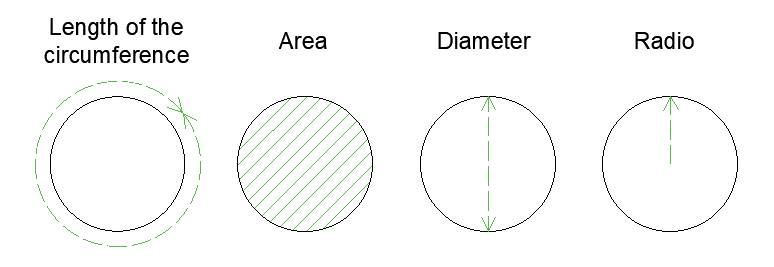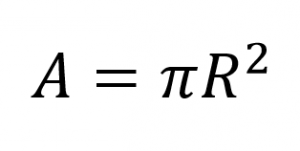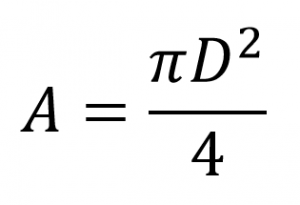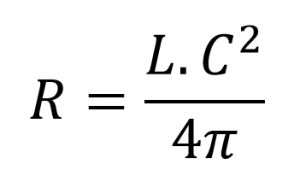# Area Calculator for automatic circle and circumference online

With this calculator you can easily know the area of ​​a circle or circumference from several variables, such as: Radius, Diameter or Length of the circumference.

In addition to the calculator you will find 3 different ways to calculate the area of ​​a circle and many practical examples.## Formulas to calculate the area of ​​a circle:

1. Formula to calculate area of ​​a circle from the radius: To make this calculation you must have the radius of the circle and with the following formula:Where:

• A: Area of ​​the circle
• π: Constant Number equivalent to 3.1415

2. How to calculate the area of ​​a circle when you have the diameter: For this calculation you must have the diameter of the circle and then enter it in the following formula:Where:

• A: Area of ​​the circle
• π: Constant Number equivalent to 3.1415
• D: Diameter of the circle

3. Finally, there is the formula for calculation of Area from the length of the circumference:  This formula facilitates the calculation of the area when you count with the length of the circumference.Where:

• π: Constant Number equivalent to 3.1415
• LC: Length of the circumference.

## Examples of use for formulas for calculating the area:

Example 1: A pizza has a radius of 20cm. What is the area of ​​the pizza?

Solution:

We take the formula from radio to area and replace the value of the radius in the following way: A = πx20cm ^ 2, the result will be: 31,83cm ^ 2

Example 2: The rim of a Mazda 2 car has a diameter of 16 “. What is the area of ​​this tire?

From the formula of diameter to area, we can replace the diameter in this formula, being as follows:  A = (πx16 “^ 2) / 4, the result will be: 20.37” ^ 2

Example 3: The pipe of an aqueduct has a circumference length of 34cm. What will be the area of ​​the pipe?

To calculate the area you must enter the value of the circumference length in the corresponding formula, as I show below:  A = (34cm ^ 2) / πx4, the result will be: 91,99cm ^ 2

[kkstarratings]